# Describing Databases with MetaData – SQLAlchemy

• Last Updated : 07 Dec, 2021

In this article, we are going to see how to describe Databases with MetaData using SQLAlchemy in Python.

Database Metadata describes the structure of the database in terms of Python data structures. The database usually consists of Tables and Columns. The Database Metadata serves us in generating SQL queries and Object Relational Mapping. It helps us in generating a Schema. The most fundamental objects of Database MetaData are MetaData, Table, and Column.

## Describing Databases with MetaData: SQLAlchemy Core

### Setting up MetaData with Table objects:

The queries in a relational database are usually created on a Table of a database. These tables are represented as Table objects in Python SQLAlchemy named as Table. The MetaData is an object which consists of Table objects keyed to their string names. The syntax of creating a MetaData object is as below:

```from sqlalchemy import MetaData

A single MetaData object is enough for an entire application. Once the MetaData object is created, we can declare Table objects. Let us look at an example of creating a table for a student account which consists of columns -name, age, and grade and we also add an id as a primary key.

We now convert the above table into a Schema by using the MetaData object.

## Python3

 `from` `sqlalchemy ``import` `MetaData``from` `sqlalchemy ``import` `Integer, String, Column, Table` `metadata_object``=``MetaData()` `student_table ``=` `Table(``     ``"student_account"``,``     ``metadata_object,``     ``Column(``'id'``, Integer, primary_key``=``True``),``     ``Column(``'name'``, String(``30``)),``     ``Column(``'age'``,Integer),``     ``Column(``'grade'``, String(``80``))``)`

In the above code, A Table represents a database table that assigns itself to the MetaData object

A Column represents a Column of a Table that assigns itself to the Table object. The Column usually contains a string name and a type object such as Integer, String, etc.

## Python3

 `from` `sqlalchemy ``import` `create_engine``from` `sqlalchemy ``import` `DateTime, Numeric, Enum` `item_detail ``=` `Table(``    ``"items"``,``    ``metadata_object,``    ``Column(``"key"``, String(``50``), primary_key``=``True``),``    ``Column(``"timestamp"``, DateTime),``    ``Column(``"price"``, Numeric(``100``, ``2``)),``    ``Column(``"type"``, Enum(``"dry"``, ``"wet"``)),``)` `# creating an engine object``engine ``=` `create_engine(``"sqlite+pysqlite:///:memory:"``,``                       ``echo``=``True``, future``=``True``)` `# emitting DDL``metadata_object.create_all(engine)`

## Accessing Tables and Columns

The columns of a Table are usually stored in an associative array i.e., Table.c, and can be accessed using “c” as shown in the following examples.

Getting table name:

## Python3

 `student_table.name`

Output:

`'student_account'`

Accessing columns using operator c:

## Python3

 `student_table.c.name`

Output:

Column(‘name’, String(length=30), table=<student_account>)

Accessing name of a column(name):

## Python3

 `student_table.c.name.name`

Output:

`name`

Accessing type of a column:

## Python3

 `student_table.c.name.``type`

Output:

`String(length=30)`

Getting the primary key of a table:

## Python3

 `student_table.primary_key`

Output:

PrimaryKeyConstraint(Column(‘id’, Integer(), table=<student_account>, primary_key=True, nullable=False))

## Accessing tables and keys using MetaData object

The metadata object can be used to access all the tables stored in the metadata object  as shown in the below examples:

## Python3

 `metadata_object.tables`

Output:

FacadeDict({‘student_account’: Table(‘student_account’, MetaData(), Column(‘id’, Integer(), table=<student_account>, primary_key=True, nullable=False), Column(‘name’, String(length=30), table=<student_account>), Column(‘age’, Integer(), table=<student_account>), Column(‘grade’,  String(length=80), table=<student_account>), schema=None), ‘items’: Table(‘items’, MetaData(),  Column(‘key’, String(length=50), table=<items>, primary_key=True, nullable=False), Column(‘timestamp’, DateTime(), table=<items>), Column(‘price’, Numeric(precision=100, scale=2), table=<items>), Column(‘type’, Enum(‘dry’, ‘wet’), table=<items>), schema=None)})

Accessing keys of tables:

## Python3

 `metadata_object.tables.keys()`

Output:

`dict_keys(['student_account', 'items'])`

## Declaring Constraints

As you can see we have declared the first Column as a  primary key in the student_table. Running the following command will show the details of the primary key constraint.

## Python3

 `student_table.primary_key`

Output:

PrimaryKeyConstraint(Column(‘id’, Integer(), table=<student_account>, primary_key=True, nullable=False))

A primary key constraint is normally declared implicitly whereas a ForeignKeyConstraint is declared explicitly. We use foreign key constraints if two tables are related to each other by using the object ForeignKey.

Let us create a new Table named address_table which consists of the email address of the student and we will have a foreign key constraint that refers to the student table.

## Python3

 `from` `sqlalchemy ``import` `ForeignKey` `address_table ``=` `Table(``     ``"address"``,``     ``metadata_object,``     ``Column(``'id'``, Integer, primary_key``=``True``),``     ``Column(``'student_id'``, ForeignKey(``'student_account.id'``), nullable``=``False``),``     ``Column(``'email_address'``, String, nullable``=``False``)`` ``)`

## Creating and Dropping Tables

### Creating Table:

So far we have created two Tables with a set of Columns and constraints. The next thing is we have to emit DDL to the SQLite database (in this case) so that we can query with the tables. This can be done as shown below:

## Python3

 `from` `sqlalchemy ``import` `create_engine` `# creating an engine object``engine ``=` `create_engine(``"sqlite+pysqlite:///:memory:"``,``                       ``echo``=``True``, future``=``True``)``# emitting DDL``metadata_object.create_all(engine)`

Output: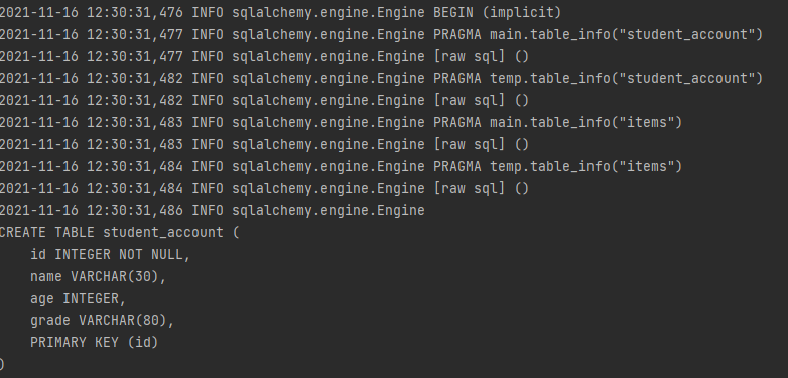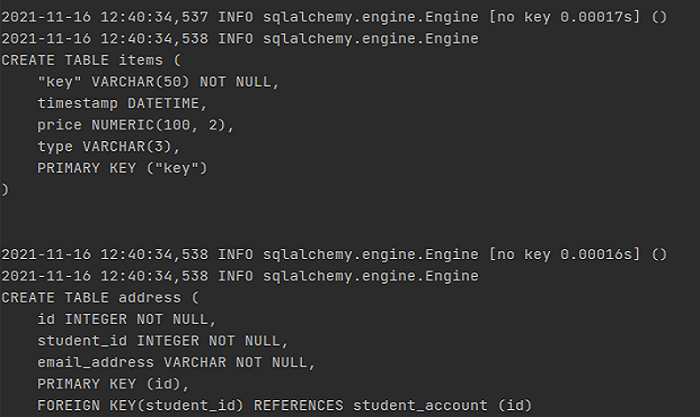### Dropping Table

The drop_all() method is used to drop all the tables in the metadata object.

## Python

 `from` `sqlalchemy ``import` `create_engine` `# creating an engine object``engine ``=` `create_engine(``"sqlite+pysqlite:///:memory:"``,``                       ``echo``=``True``, future``=``True``)` `# emitting DDL``metadata_object.drop_all(engine)`

Output: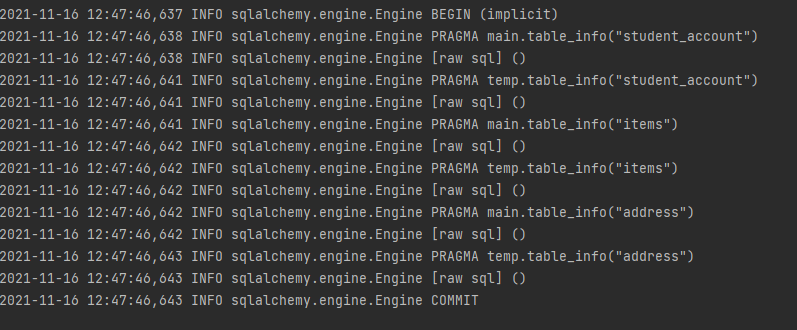## Describing Databases with MetaData: SQLAlchemy ORM

We will learn how to create the same tables as we have created using Core with ORM. The ORM consists of mapped classes. The mapped classes are declared in the same manner as any Python class is created and we link the attributes of the mapped classes to the Columns of the Table.

In ORM the MetaData object is combined with the ORM-Only object called Registry. We construct the Registry as shown below:

## Python3

 `from` `sqlalchemy.orm ``import` `registry` `mapper_registry ``=` `registry()``mapper_registry.metadata`

Output:

`MetaData()`

In ORM, instead of declaring Table objects directly, we declare them indirectly by using mapped classes. The mapped classes descend from a base directory known as Declarative Base. The declarative base can be created using registry using the registry.generate_base() method.

## Python3

 `Base ``=` `mapper_registry.generate_base()`

This base class serves as the base class for the ORM mapped classes that we declare. The two tables Student_account and address can be created using ORM as shown below.

## Python3

 `from` `sqlalchemy.orm ``import` `relationship`  `class` `Student(Base):``    ``__tablename__ ``=` `'student_account'``    ``id` `=` `Column(Integer, primary_key``=``True``)``    ``name ``=` `Column(String(``30``))``    ``age ``=` `Column(Integer)``    ``grade ``=` `Column(String)` `    ``addresses ``=` `relationship(``"Address"``, back_populates``=``"student"``)` `    ``def` `__repr__(``self``):``        ``return` `f"Student(``id``=``{``self``.``id``!r}, name``=``{``self``.name!r},\``        ``age``=``{``self``.age!r},grade``=``{``self``.grade!r})"`  `class` `Address(Base):``    ``__tablename__ ``=` `'address'``    ``id` `=` `Column(Integer, primary_key``=``True``)``    ``email_address ``=` `Column(String, nullable``=``False``)``    ``student_id ``=` `Column(Integer, ForeignKey(``'student_account.id'``))` `    ``student ``=` `relationship(``"Student"``, back_populates``=``"addresses"``)` `    ``def` `__repr__(``self``):``        ``return` `f``"Address(id={self.id!r}, email_address={self.email_address!r})"`

The .__table__ attribute is used to see the Table objects created from declarative mapped classes as shown below:

## Python3

 `Student.__table__`

Output:

```Table('student_account', MetaData(), Column('id', Integer(),
table=<student_account>, primary_key=True, nullable=False),
Column('name', String(length=30), table=<student_account>),
Column('age', Integer(), table=<student_account>),

## Python3

 `Address.__table__`

Output:

```Table('address', MetaData(), Column('id', Integer(),
nullable=False), Column('student_id', Integer(),

### Emitting DDL:

In ORM, for emitting DDL we use the MetaData object associated with our registry and ORM declarative base class.

## Python3

 `mapper_registry.metadata.create_all(engine)` `Base.metadata.create_all(engine)`

Output: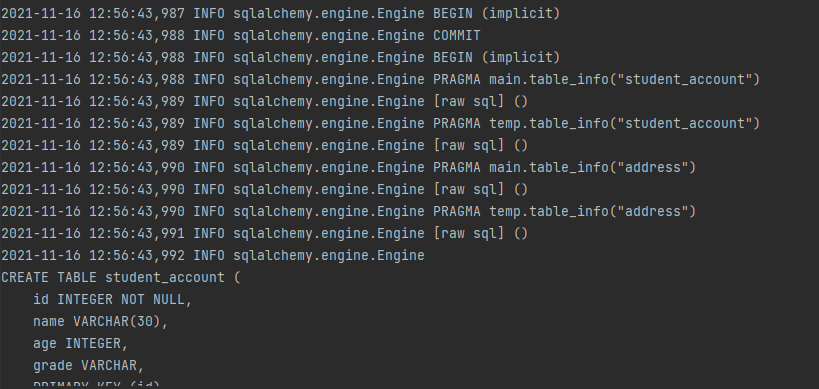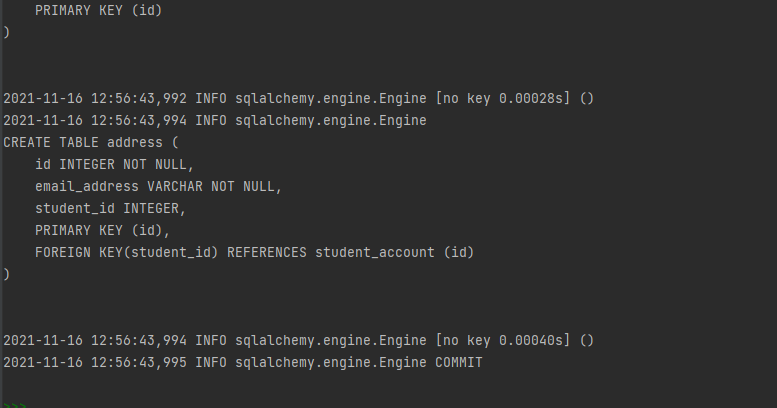## Table Reflection

“Reflection” refers to loading Table objects based upon reading from an existing Table. For example, we will create a new Table object which represents the student_account object we created manually in the earlier sections of this article as below.

## Python3

 `metadata2``=``MetaData()``with engine.connect() as conn:``    ``student_reflected``=``Table(``"student_account"``,``                            ``metadata2,``                            ``autoload_with``=``conn)`

Output: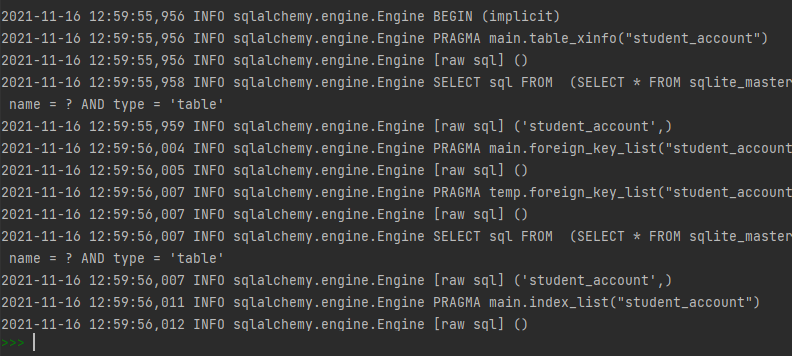You can now access the columns of the reflected table we just created as follows :

## Python3

 `print``(student_reflected.c)``print``(student_reflected.primary_key)`

Output: